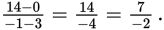# SAT Math Multiple Choice Question 523: Answer and Explanation

### Test Information

Question: 523

 x 3 –1 –5 –7 y 0 14 28 ?

13. If the values in the table represent a linear relationship, what is the missing value?

• A. 21
• B. 30
• C. 35
• D. 42

Getting to the Answer: Choose any two points (preferably ones with the nicest numbers) from the table, and substitute them into the slope formula. Using the points (3, 0) and (–1, 14), the slope isThis means that for every 2 units the x-value decreases, the y-value increases by 7, and the decrease from x = –5 to x = –7 happens to be –2. So, increase the y-value by 7 one time: 28 + 7 = 35.